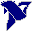# LabVIEW

cancel
Showing results for
Search instead for
Did you mean:

Solved!
Go to solution

## Question on FPGA IP Builder Example

Hi,

In the FPGA IP Builder example attached, In the CORDIC VI why are the values of x, y and z limited to <1, also how are the ztable array values selected.

As per my understanding of CORDIC z' = z - (di)(angle)

where angle is selected such that, angle = atan 2^i

and i is the iteration count.

di is the decision factor.

But this is not the case in the example presented.

I am trying to understand how High throuhput Natural logarithm works, and after reading about it I have come to a conclusion that it uses CORDIC so I am trying understand CORDIC and how it is implemented in FPGA.

Message 1 of 7
(231 Views)

## Re: Question on FPGA IP Builder Example

Thank you for posting and asking the question "What is the CORDIC Algorithm" (I could see its implementation in your code, but had no idea what it was really doing ...).

So I looked it up!  Fascinating.  CORDIC is an acronym for COordinate Rotation DIgital Computer, which uses a series of shifts, simple logic (basically sign of the intermediate result) and an extremely clever way of building up an arbitrary rotation angle (angles are chosen such that their tangents are well-approximated by a shift, i.e. they represent a power of 2).  Using this, it is really fast (and needs almost no "fancy computation") to express functions such as sin(x) or arctan(x).

Here's one site that develops the idea:  An Introduction to the CORDIC Algorithm .

Bob Schor

Message 2 of 7
(160 Views)

## Re: Question on FPGA IP Builder Example

Thank you for your response.

I am aware of the mathematical aspect of CORDIC algorithm and its applications. My question was how is it implemented in Labview FPGA inside a SCTL, as it is iterative I don't think it is possible to implement it without pipeline. Also the code that I attached is an example code from Labview 2019.

The values of z' in cordic are selected in cordic such that z' = zi - di * atan(2^-i)

where zi and di refer to the ith iteration. However the values present in the ztable array in the given example do not correspond to atan(2^-i), which according to me should be 45, 26.56, 14.036, 7.12, 3.576 and so on for i = 0,1,2,3,4,...

Message 3 of 7
(131 Views)

## Re: Question on FPGA IP Builder Example

Ah.  That I understand.  For the algorithm to work, you need to have representations of atan(1), atan(0.5), atan(0.25), atan(0.125), etc.  The values in the table are simply 2^14 (=16384) * [atan(1), atan(0.5), atan(0.25), atan(0.125), ...].  Note that multiplying by a power of 2 in an FPGA amounts to a bit shift -- can you figure out why you shift by 14?  [Hint -- it has to do with maintaining maximum precision without worrying about a sign bit].

Bob Schor

Message 4 of 7
(120 Views)

## Re: Question on FPGA IP Builder Example

Unfortunately that is not the case here. I had already considered this possibility.

You see the values in the table are 16384, 9672, 5110, 2594, 1302, 652, 326, 163, 81, 41, 20, 10, 5, 3, 1, 1

Whereas according to the logic you mentioned the values should have been 16384, 8192, 4096, 2048, 1024, and so on which is not the case....

Message 5 of 7
(76 Views)
Solution
Accepted by topic author linu95k

## Re: Question on FPGA IP Builder Example

Hi linu,

@linu95k wrote:

However the values present in the ztable array in the given example do not correspond to atan(2^-i), which according to me should be 45, 26.56, 14.036, 7.12, 3.576 and so on for i = 0,1,2,3,4,...

You see the values in the table are 16384, 9672, 5110, 2594, 1302, 652, 326, 163, 81, 41, 20, 10, 5, 3, 1, 1

Let's see:

45.00 is represented by 16384

26.56 is represented by 9672: 26.56745*16384=9670.2

14.04 is represented by 5110: 14.04/45*16384=5110.8

7.12 is represented by 2594: 7.12/45*16384=2592.3

Do you see the pattern? (Apart from rounding errors…)

Best regards,
GerdWusing LV2011SP1 + LV2017 (+LV2020 sometimes) on Win10+cRIO
Message 6 of 7
(70 Views)

## Re: Question on FPGA IP Builder Example

Yes, I understand now. Thank you very much for the help.

Message 7 of 7
(61 Views)